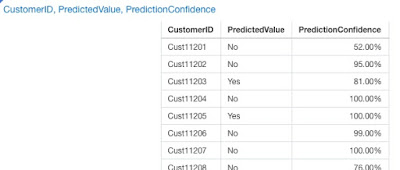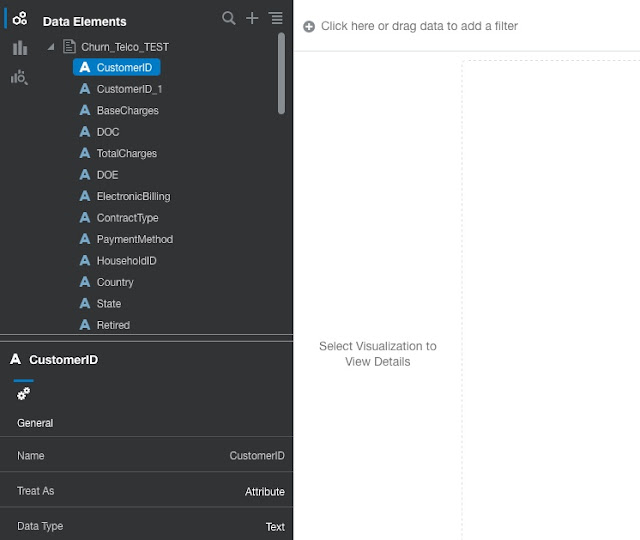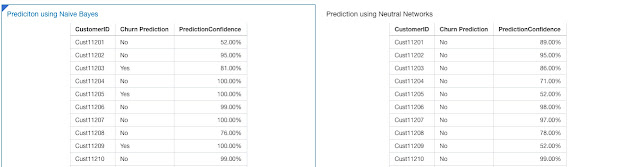ShareORACLE DATA VISUALIZATION AND MACHINE LEARNING

It's been a while since my last post. Since then we have been playing with the latest versions of Oracle Data Visualization Desktop and Oracle Analytic Cloud. I must admit that Oracle has made a significant progress with the DV tools.One of the key developments was made in the area of Machine Learning, which has been added just recently and brings machine learning algorithms closer to users. End users can actually deploy very complex algorithms with just "a click of the button".

In today's post I am playing with preparing data for machine learning, creating machine learning models with different machine learning algorithms and finally applying those to new data sets in order to predict churn.

DATA

I used a data set from Kaggle (https://www.kaggle.com/hkalsi/telecom-company-customer-churn/data).As you can see data set is split into 4 csv files that have to be merged into one training and one test data set. Actually, the test data set is in fact contains a data set for customer which still need prediction, so it is not really a test data set.

Files Train.csv, Train_AccountInfo.csv and Train_Demographincs.csv all contain 5298 rows.  However Train_ServicesOptedFor.csv contains 47683 rows. Brief investigation shows that this table needs to be pivoted. With pivoting transformation and applying one-hot encoding Train_Services_pivoted.csv contains 5298.

This same transformation has to be run over Test_ServicesOptedFor.csv.

At the moment, for this instance this transformation has to be done outside Data Visualization tool.

USING DATA VISUALIZATION DATA FLOWS TO CREATE A NEW DATA SET

With Data Flows users can create a data flow that merges all 4 data files into one single data set. Train data set contains merged records, one row per customer, including target column of Churn.CREATING A NEW MACHINE LEARNING MODEL

Data Flows are used to create a new Machine Learning model. There are specific steps, operators, that can be used for training the model using :

- numeric prediction,
- binary classifier,
- multi-classifier,
- clustering,
- custom Model.

Churn prediction is an example of binary classifier because there are only two options available, customer has churned (Churn value is Yes) or customer has not churned (Churn value is No).

Data Flow which creates a new machine learning model has 3 steps in which data set is read, a data model is created and stored.The most important step is obviously the one in the middle, where machine learning model is created. So let's take a look at it a bit closer.

In our first example, we are using Naive Bayes binary classifier to train our model.

##There are several attributes that you need to set before you execute data flow and model is created.

The mandatory parameter is the Target. This is the attribute we are predicting, the Churn. And in this case we have 2 values to predict, Yes and No. Yes in our case is also treated as positive outcome (hmm, would it be better No? - actually it doesn't matter that much at the moment).

Missing values have to be handled before any algorithm is run. There are several strategies how to resolve missing values. In the case above, most frequent value and mean in the dataset will replace missing values for a particular attribute, depending on attribute type - Categorical or Numeric. Another preparatory step is also to encode categorical values, which means replacing labels with an index or even better (using one-hot encoding) to replace a categorical attribute value with set of "binary" code. This is important as some algorithms would expect only values between 0 (or -1) and 1.

At the end it is very important to know, that the training data set needs to be split into two parts. The first one, in example above 80% of all row/instances, would be used to train the model and the remaining part would be used for testing it. 100% simply can not be used as the model could be over fitted. It would work 100% correct for the training data set, but it could miserably fail for any other data set.

EVALUATING THE MODEL

Once the data flow is created and executed, a new Machine Learning model is placed in the list of available models.You can always inspect any model. This will give you key information of  how good the model is performing.From the confusion matrix on the right, we can derive some of the measures or metrics that explain the quality of the model that was created. Confusion matrix is a table with 2 dimensions: Actual and Predicted values. Each row of the matrix represents the instances in a predicted class while each column represents the instances in an actual class.

Precision (positive predictive value) is the fraction of relevant instances among the retrieved instances, while recall (sensitivity) is the fraction of relevant instances that have been retrieved over the total amount of relevant instances. The following formulas can be used to calculate Precision and Recall.

Precision = number of "true-positives" instances / (number of "true-positive" instances + number of "false positive" instances)

Recall = number of "true-positive" instances / (number of "true-positive" instances + number of "false negative" instances)

F1 Value is a measure of test's accuracy. It is a combination of precision and recall and is calculated using the following formula:

F1 = 2 x (Precision x Recall) / (Precision + Recall)

The F1 Value is the harmonic average of the precision and recall, where an F1 Value reaches its best value at 1 and worst at 0. In the case above, F1 value is a bit over an average.

Model accuracy is a measure of how well a binary classification test correctly identifies or excludes a condition. That is, the accuracy is the proportion of true results (both true positives and true negatives) among the total number of cases examined.

Model accuracy =  (number of "true-positive" instances + number of "true-negative" instances) / (number of all instances)

This way we can see that precision in the model just created is not very high, whereas recall is. F1 Value is a bit better than average. Accuracy is also not very high as well.

Selection of some other model might give us better results. For example model build using Neural Network gives us better model accuracy and precision. Recall is definitely worse. False positive rate is also reduced. But after all, we can conclude that Neutral Network performs better than Naive Bayes classifier. At least in this case.APPLYING THE MODEL

Now we are ready to apply a model. One way of doing it is to create another data flow.Input data set is a new data set which doesn't have Churn attribute. Not just yet. As this is our target in the model, it will be generated based on the machine learning model. A new data set will actually contain two new attributes, the predicted churn value and the confidence in predicted value.Alternative way of applying generated machine learning model is to use so-called Scenarios.

In this case, start with a test data set (the one without target attribute) and create a new project.

##Click on "+" and select Create Scenario from the menu list.List of available Machine Learning Models is display. You can select and add any number of models. Once selected they will be added to the Data Element list, from where you can freely add Prediction Value and Prediction Confidence attributes to the analysis.You can check the results of the two models. There were some differences to be expected based on the evaluation of the two models.In this case, machine learning is applied directly on the data set within the analysis and no extra "churn predicted" data set is required.  This could save us some time. Of course in case of long-running prediction algorithms, using data flows to create a predicted data set seems to be the only viable option.

But it is still cool, especially if you are analyst who knows what to expect but doesn't have any idea how to write R or Python code.

CONCLUSION

Oracle Data Visualization products have been significantly improved over the last couple of month, in particular in Machine Learning support. As you can see there are already a number of prebuilt machine learning algorithms, but the nice thing about this is also possibility to create your own algorithms using R or Python (who says Data Scientist will no longer be needed!). I am sure many of user would prefer this. And on the other hand side if these custom algorithms were tested and moved into production, then end users could simply use them, not dealing with what is actually behind the scenes. I guess very compelling story. Isn't it?

Become a member

### Newsletter

Receive our newsletter to stay on top of the latest posts.